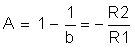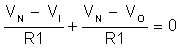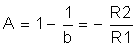SLOA011B January   2018  – July 2021

1. 1Introduction
2. 2Non-Inverting Amplifier
3. 3Inverting Amplifier
4. 4Simplified Op Amp Circuit Diagram
5. 5Op Amp Specifications
6. 6References
7. 7Glossary
8. 8Revision History

## 3.1 Closed Loop Concepts and Simplifications

Substituting a = ∞ Equation 1 into Equation 24 results in

Equation 27.Recall that in equation Equation 6 we stated that Vd, the voltage difference between Vn and Vp, was equal to zero so that Vn = Vp. Still they are not shorted together. Rather there is said to be a virtual short between Vn and Vp. The concept of the virtual short further simplifies analysis of the inverting op amp circuit in Figure 3-1.

Using the virtual short concept, we can say that

Equation 28. Vn = Vp = 0

In this configuration, the inverting input is a virtual ground.

We can write the node equation at the inverting input as

Equation 29.Since Vn = 0, rearranging, and solving for A we get

Equation 30.The same result is derived more easily than in (Equation 24. Using the virtual short (or virtual ground) concept reduced solving the inverting amplifier, shown in Figure 3-1, to solving a single node equation.